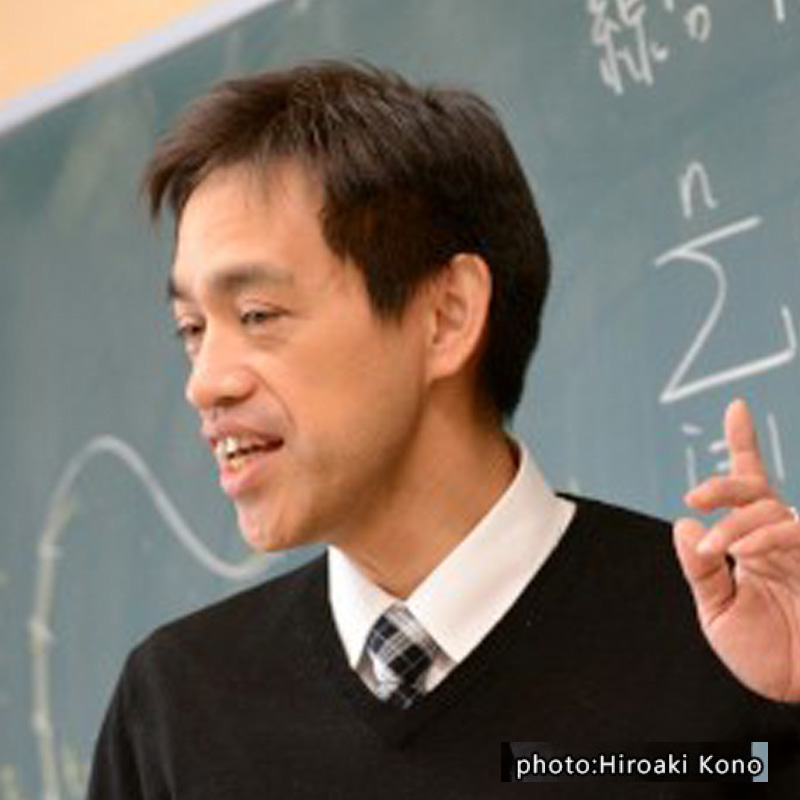## KUBO HideoProfessor

### Read natural phenomena by mathematics

Department of Mathematics, MathematicsTheme It is quite exciting to understand universal structure through analysis of mathematical models, such as partial differential equations. Field Theory of differential equations, Applied mathematics Keyword Nonlinear wave, Asymptotic analysis, Self-organization

#### Introduction of Research